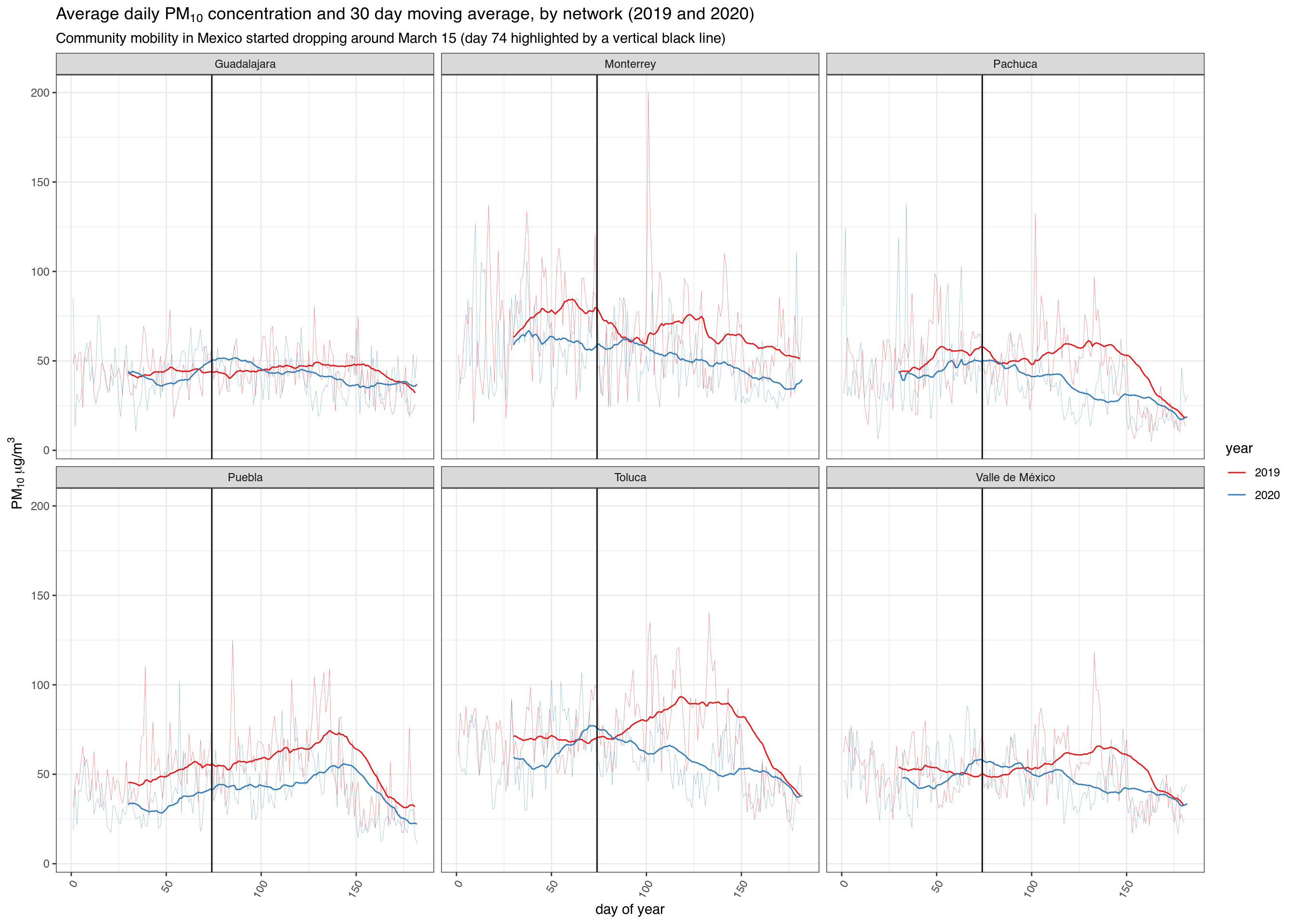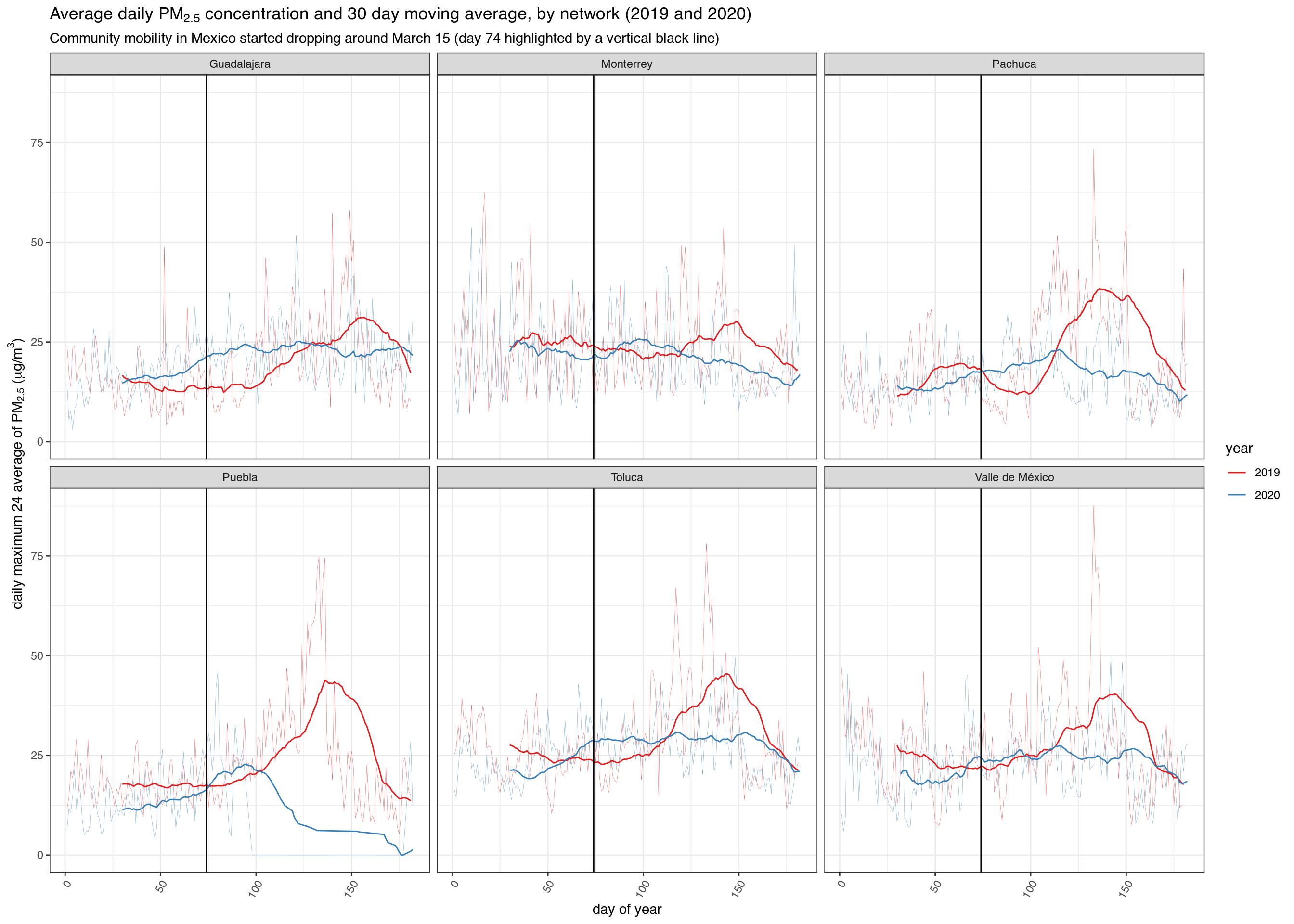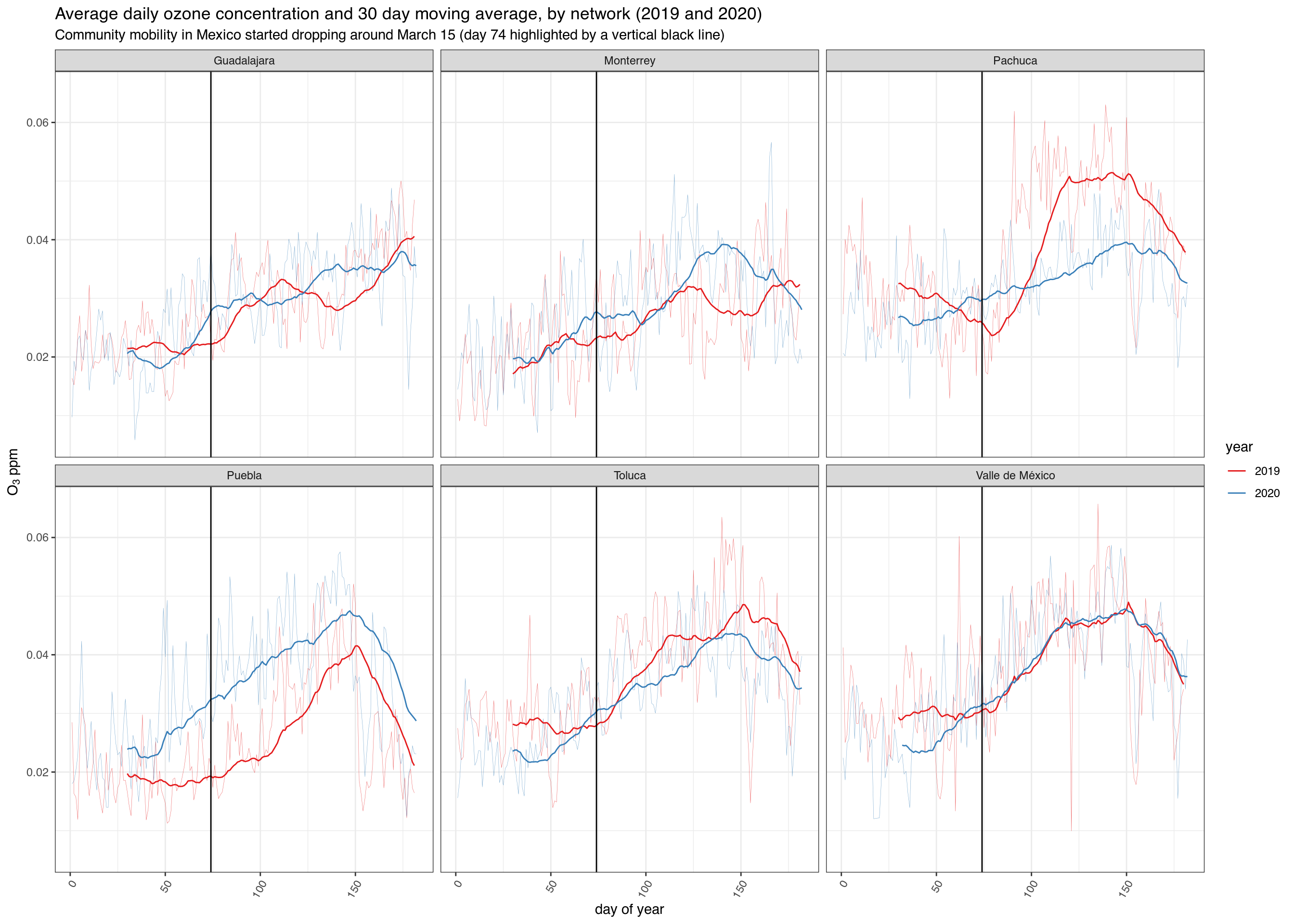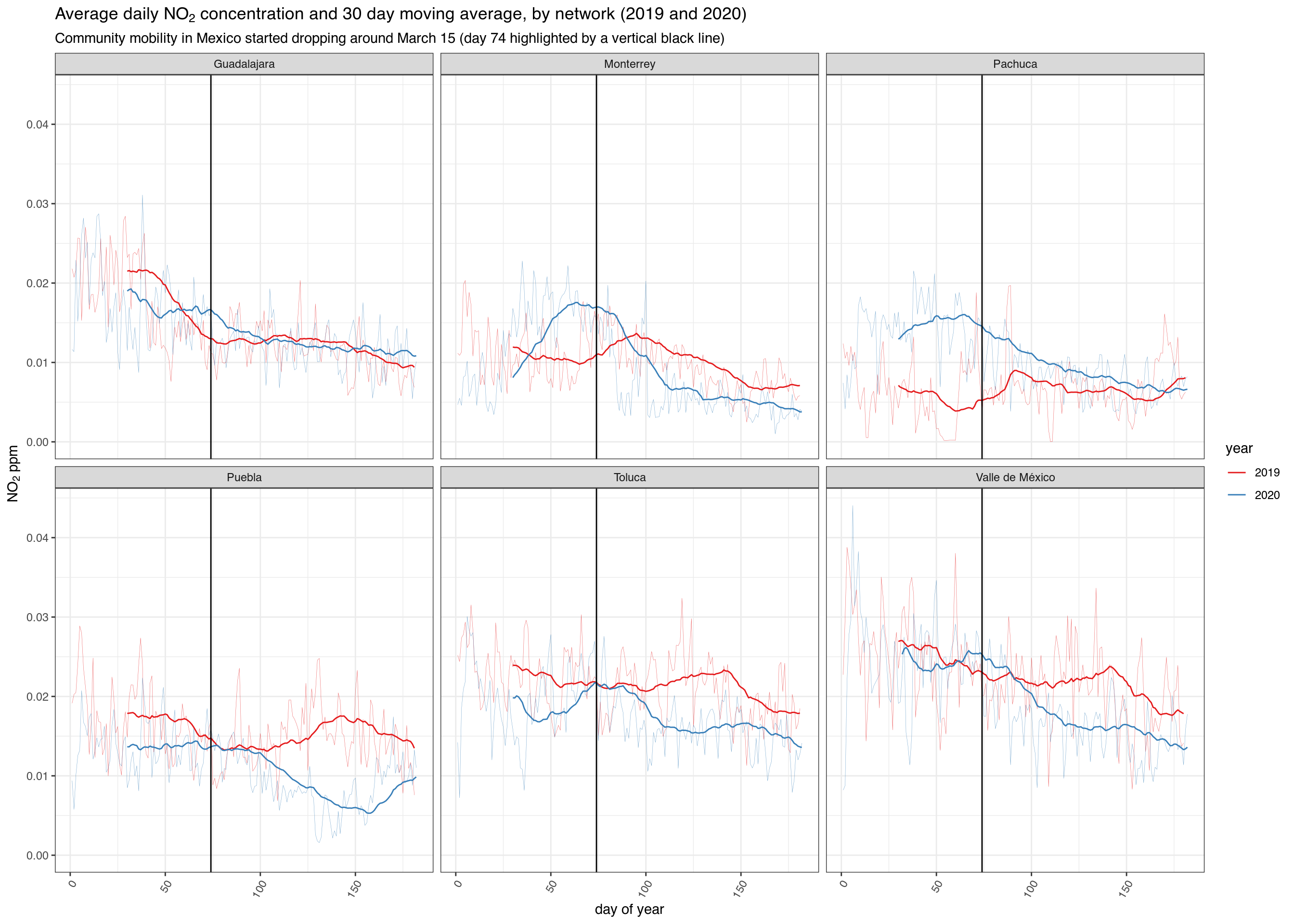## COVID and Air Pollution in Mexico

We can use rsinaica to find out what happened to air pollutions levels in several Mexican cities after people started taking social distancing measures due to the novel coronavirus

First, we load the packages necessary for the analysis

## Auto-install required R packages
packs <- c("dplyr", "ggplot2", "gghighlight", "lubridate", "anomalize",
"aire.zmvm", "tidyr", "zoo", "plotly", "rsinaica", "ggseas")
success <- suppressWarnings(sapply(packs, require, character.only = TRUE))
if (length(names(success)[!success])) {
install.packages(names(success)[!success])
sapply(names(success)[!success], require, character.only = TRUE)
}

Then we download the data for the whole 2020 and 2019 using the sinaica_param_data function. Since the maximum data range we can download is 1 month, we have to use a little mapply magic to download the entire year.

# Download all PM10 pollution data in 2017
pm10_2020 <- bind_rows(
mapply(sinaica_param_data,
"PM10",
seq(as.Date("2020-01-01"), as.Date("2020-06-01"), by = "month"),
seq(as.Date("2020-02-01"), as.Date("2020-07-01"), by = "month") - 1,
remove_extremes = FALSE,
SIMPLIFY = FALSE)
)
pm10_2019 <- bind_rows(
mapply(sinaica_param_data,
"PM10",
seq(as.Date("2019-01-01"), as.Date("2019-06-01"), by = "month"),
seq(as.Date("2019-02-01"), as.Date("2019-07-01"), by = "month") - 1,
remove_extremes = FALSE,
SIMPLIFY = FALSE)
)

This is what the data looks like:

knitr::kable(head(pm10_2020))
id station_id station_name station_code network_name network_code network_id date hour parameter value_original flag_original valid_original value_actual valid_actual date_validated validation_level unit value
101PM1020010100 101 Atemajac ATM Guadalajara GDL 63 2020-01-01 0 PM10 58.326 128 1 58.326 1 NA 0 µg/m³ 58.326
101PM1020010101 101 Atemajac ATM Guadalajara GDL 63 2020-01-01 1 PM10 104.09 128 1 104.09 1 NA 0 µg/m³ 104.090
101PM1020010102 101 Atemajac ATM Guadalajara GDL 63 2020-01-01 2 PM10 101.73 128 1 101.73 1 NA 0 µg/m³ 101.730
101PM1020010103 101 Atemajac ATM Guadalajara GDL 63 2020-01-01 3 PM10 90.637 128 1 90.637 1 NA 0 µg/m³ 90.637
101PM1020010104 101 Atemajac ATM Guadalajara GDL 63 2020-01-01 4 PM10 82.158 128 1 82.158 1 NA 0 µg/m³ 82.158
101PM1020010105 101 Atemajac ATM Guadalajara GDL 63 2020-01-01 5 PM10 95.17 128 1 95.17 1 NA 0 µg/m³ 95.170

The data is hourly, but we can average it to the daily levels and filter it to only include major cities. Obviously a more formal analysis would make sure that no new stations were added during the periods we are comparing and would filter the measurement errors that are sometimes returned by the sensors.

# Only include stations that reported for more than 80% of days
df_filtered <- bind_rows(pm10_2019, pm10_2020) %>%
filter(!is.na(value)) %>%
filter(!(value > .0955 & hour %in% c(0:11, 21:23))) %>%
filter(value < 300) %>%
filter(n > 180*.8)  %>%
select(-n)

df_ave <- df_filtered %>%
group_by(date, network_name) %>%
summarise(ave = mean(value, na.rm = TRUE), .groups = 'drop') %>%
arrange(network_name) %>%
mutate(year = year(date)) %>%
mutate(doy = yday(date)) %>%
filter(network_name %in% c("Valle de México", "Monterrey", "Toluca",
"Guadalajara", "Pachuca", "Puebla"))
ggplot(df_ave, aes(doy, ave, group = year, color = as.factor(year))) +
geom_line(size = .1, alpha = .7) +
scale_color_brewer("year", type='qual', palette = "Set1") +
facet_wrap(~ network_name, ncol = 3) +
ggtitle(expression(paste("Average daily ", PM, " concentration and 30 day moving average, by network (2019 and 2020)"))) +
labs(subtitle = "Community mobility in Mexico started dropping around March 15 (day 74 highlighted by a vertical black line)") +
xlab("day of year") +
ylab(expression(paste(PM, " ", mu,"g/", m^3))) +
theme_bw() +
stat_rollapplyr(width = 30, align = "right") +
geom_vline(xintercept = 74) + #March 15 is day number 74
theme(axis.text.x=element_text(angle=60,hjust=1))
## Warning: Removed 58 row(s) containing missing values (geom_path).## PM2.5

# Download all PM10 pollution data in 2017
pm25_2020 <- bind_rows(
mapply(sinaica_param_data,
"PM2.5",
seq(as.Date("2020-01-01"), as.Date("2020-06-01"), by = "month"),
seq(as.Date("2020-02-01"), as.Date("2020-07-01"), by = "month") - 1,
remove_extremes = FALSE,
SIMPLIFY = FALSE)
)
pm25_2019 <- bind_rows(
mapply(sinaica_param_data,
"PM2.5",
seq(as.Date("2019-01-01"), as.Date("2019-06-01"), by = "month"),
seq(as.Date("2019-02-01"), as.Date("2019-07-01"), by = "month") - 1,
remove_extremes = FALSE,
SIMPLIFY = FALSE)
)
# Only include stations that reported for more than 80% of days
df_filtered <- bind_rows(pm25_2019, pm25_2020) %>%
filter(!is.na(value)) %>%
filter(!(value > .0955 & hour %in% c(0:11, 21:23))) %>%
filter(n > 180*.8)  %>%
select(-n)

df_ave <- df_filtered %>%
group_by(date, network_name) %>%
summarise(ave = mean(value, na.rm = TRUE), .groups = 'drop') %>%
arrange(network_name) %>%
mutate(year = year(date)) %>%
mutate(doy = yday(date)) %>%
filter(network_name %in% c("Valle de México", "Monterrey", "Toluca",
"Guadalajara", "Pachuca", "Puebla"))
ggplot(df_ave, aes(doy, ave, group = year, color = as.factor(year))) +
geom_line(size = .1, alpha = .7) +
scale_color_brewer("year", type='qual', palette = "Set1") +
facet_wrap(~ network_name, ncol = 3) +
ggtitle(expression(paste("Average daily ", PM[2.5], " concentration and 30 day moving average, by network (2019 and 2020)"))) +
labs(subtitle = "Community mobility in Mexico started dropping around March 15 (day 74 highlighted by a vertical black line)") +
xlab("day of year") +
ylab(expression(paste("daily maximum 24 average of ", PM[2.5],
" (", mu,"g/", m^3, ")"))) +
theme_bw() +
stat_rollapplyr(width = 30, align = "right") +
geom_vline(xintercept = 74) + #March 15 is day number 74
theme(axis.text.x=element_text(angle=60,hjust=1))
## Warning: Removed 58 row(s) containing missing values (geom_path).## O3

# Download all PM10 pollution data in 2017
o3_2020 <- bind_rows(
mapply(sinaica_param_data,
"O3",
seq(as.Date("2020-01-01"), as.Date("2020-06-01"), by = "month"),
seq(as.Date("2020-02-01"), as.Date("2020-07-01"), by = "month") - 1,
remove_extremes = FALSE,
SIMPLIFY = FALSE)
)
o3_2019 <- bind_rows(
mapply(sinaica_param_data,
"O3",
seq(as.Date("2019-01-01"), as.Date("2019-06-01"), by = "month"),
seq(as.Date("2019-02-01"), as.Date("2019-07-01"), by = "month") - 1,
remove_extremes = FALSE,
SIMPLIFY = FALSE)
)
# Only include stations that reported for more than 80% of days
df_filtered <- bind_rows(o3_2019, o3_2020) %>%
filter(!is.na(value)) %>%
filter(!(value > .0955 & hour %in% c(0:11, 21:23))) %>%
filter(value < .3) %>%
filter(n > 180*.8)  %>%
select(-n)

df_ave <- df_filtered %>%
group_by(date, network_name) %>%
summarise(ave = mean(value, na.rm = TRUE), .groups = 'drop') %>%
arrange(network_name) %>%
mutate(year = year(date)) %>%
mutate(doy = yday(date)) %>%
filter(network_name %in% c("Valle de México", "Monterrey", "Toluca",
"Guadalajara", "Pachuca", "Puebla"))
ggplot(df_ave, aes(doy, ave, group = year, color = as.factor(year))) +
geom_line(size = .1, alpha = .7) +
scale_color_brewer("year", type='qual', palette = "Set1") +
facet_wrap(~ network_name, ncol = 3) +
ggtitle("Average daily ozone concentration and 30 day moving average, by network (2019 and 2020)") +
labs(subtitle = "Community mobility in Mexico started dropping around March 15 (day 74 highlighted by a vertical black line)") +
xlab("day of year") +
ylab(expression(paste(O, " ppm"))) +
theme_bw() +
stat_rollapplyr(width = 30, align = "right") +
geom_vline(xintercept = 74) + #March 15 is day number 74
theme(axis.text.x=element_text(angle=60,hjust=1))
## Warning: Removed 58 row(s) containing missing values (geom_path).## NO2

# Download all PM10 pollution data in 2017
no2_2020 <- bind_rows(
mapply(sinaica_param_data,
"NO2",
seq(as.Date("2020-01-01"), as.Date("2020-06-01"), by = "month"),
seq(as.Date("2020-02-01"), as.Date("2020-07-01"), by = "month") - 1,
remove_extremes = FALSE,
SIMPLIFY = FALSE)
)
no2_2019 <- bind_rows(
mapply(sinaica_param_data,
"NO2",
seq(as.Date("2019-01-01"), as.Date("2019-06-01"), by = "month"),
seq(as.Date("2019-02-01"), as.Date("2019-07-01"), by = "month") - 1,
remove_extremes = FALSE,
SIMPLIFY = FALSE)
)
# Only include stations that reported for more than 80% of days
df_filtered <- bind_rows(no2_2019, no2_2020) %>%
filter(!is.na(value)) %>%
filter(!(value > .0955 & hour %in% c(0:11, 21:23))) %>%
filter(value < .3) %>%
filter(n > 180*.8)  %>%
select(-n)

df_ave <- df_filtered %>%
group_by(date, network_name) %>%
summarise(ave = mean(value, na.rm = TRUE), .groups = 'drop') %>%
arrange(network_name) %>%
mutate(year = year(date)) %>%
mutate(doy = yday(date)) %>%
filter(network_name %in% c("Valle de México", "Monterrey", "Toluca",
"Guadalajara", "Pachuca", "Puebla"))
ggplot(df_ave, aes(doy, ave, group = year, color = as.factor(year))) +
geom_line(size = .1, alpha = .7) +
scale_color_brewer("year", type='qual', palette = "Set1") +
facet_wrap(~ network_name, ncol = 3) +
ggtitle(expression(paste("Average daily ", NO, " concentration and 30 day moving average, by network (2019 and 2020)"))) +
labs(subtitle = "Community mobility in Mexico started dropping around March 15 (day 74 highlighted by a vertical black line)") +
xlab("day of year") +
ylab(expression(paste(NO, " ppm"))) +
theme_bw() +
stat_rollapplyr(width = 30, align = "right") +
geom_vline(xintercept = 74) + #March 15 is day number 74
theme(axis.text.x=element_text(angle=60,hjust=1))
## Warning: Removed 58 row(s) containing missing values (geom_path).